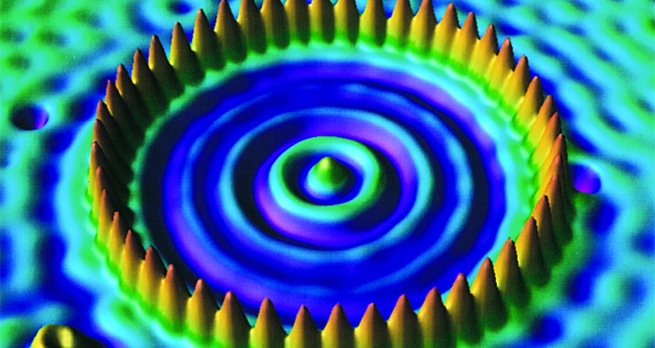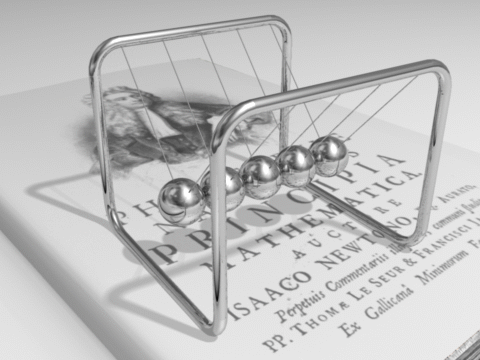Collisions and conservation laws

Start this free course now. Just create an account and sign in. Enrol and complete the course for a free statement of participation or digital badge if available.

Free course

# 3.3 Four special cases of general elastic collisions

It is interesting to examine these results for and in a few special cases, including some that have been mentioned earlier. The cases are illustrated in Animation 1, and have many familiar sporting applications.

Active content not displayed. This content requires JavaScript to be enabled.
Animation 1 Animation showing body with mass and initial speed moving in one dimension and colliding with body with mass and speed initially moving in the opposite direction. The controls in the animation allow you to change the values of , , and . Press the play button to see what happens during the elastic collision.
Interactive feature not available in single page view (see it in standard view).

## Activity 5

Using Animation 1, explore what happens in the cases listed below and find, using Equation 8 and Equation 9, expressions for and :

(a)

With the masses equal, we can see that if particle is moving slowly and approaching particle which is moving quickly, then after the collision, the particles rebound but now particle is moving quickly and particle is moving slowly. In fact, Equation 8 and Equation 9 give and so the velocities of the particles are simply exchanged.

(b)

The motion of the high-mass particle is virtually unchanged by the collision. If is very small compared with , then and . With , Equation 8 and Equation 9 then give and . The low-mass particle moves off with a velocity of twice that of the high-mass particle.

(c)

The low-mass particle rebounds with almost unchanged speed while the high-mass particle remains essentially at rest. If is very small compared with (which is stationary), then Equation 8 and Equation 9 lead to and .

(d)

The low-mass particle bounces back with higher speed, while the high-mass particle continues essentially unaffected by the collision. If is negligible compared with , and the two bodies approach head-on with equal speeds then Equation 8 and Equation 9 lead to and .

The results for the four special cases in the exercises accord with common experience. Let’s summarise them:

1. : the particles simply exchange velocities. We saw this result earlier in this section; it is a familiar occurrence in bowls and snooker.

2. ; : the low-mass particle moves off with a velocity of twice that of the high-mass particle. Tennis players will be familiar with this case from serving.

3. ; : the low-mass particle rebounds with almost unchanged speed while the high-mass particle remains essentially at rest. Golfers whose ball hits a tree will recognise this situation.

4. ; : the low-mass particle bounces back with three times the initial speed, while the high-mass particle continues essentially unaffected by the collision. This case will be recognised by a batsman playing cricket or by a tennis player returning a serve.

The results quoted above under points 2, 3 and 4 give an upper limit to the speed that can be imparted to a ball hit by a club, bat or racket.

## Activity 6

The ‘Newton’s cradle’ executive toy shown below performs repeated collisions between one ball and a row of four identical balls. You may assume the collisions are perfectly elastic.Figure 1 ‘Newton’s cradle’ executive toy: a row of four balls suspended from a frame collide in sequence

(a) Explain how this toy fits into the framework of four special cases enumerated above.

While at first glance, we have the situation ; , most closely corresponding to point 3 above, the behaviour of the toy is not as predicted by point 3. In fact, the toy executes a rapid series of four repeated collisions corresponding to point 1 above: with the case . The second ball moves instantaneously with velocity , colliding immediately with the third ball, which moves instantaneously with velocity , and so on until the fifth ball carries the momentum away with velocity .

(b) Gravitational potential energy is energy possessed by an object by virtue of its height – objects further from the surface of the Earth will have a greater gravitational potential energy. As an object falls, its gravitational potential energy will be converted into kinetic energy, and as an object rises, its kinetic energy will be converted into gravitational potential energy. What role does the exchange of gravitational potential energy and kinetic energy play in the dynamics of the first and fifth balls?

After the four collisions, the fifth ball moves in a pendulum-like trajectory until its kinetic energy is converted to gravitational potential energy. It comes instantaneously to rest, then moves back to collide with the fourth ball with velocity (neglecting air resistance encountered during the motion). The same thing happens when the first ball is set in motion by the second ball, it begins to move as a pendulum with velocity . This kinetic energy is again converted to gravitational energy until the first ball comes instantaneously to rest, then moves back to collide with the second ball with velocity , repeating the cycle.

S217_1